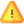Conducttr allows you to use the following mathematical operators inside Smartword Expressions:
*/+-^                        standard maths operators
LOOKUP                  tries to find a value in a list of values and returns the list position
MAX                         returns the biggest number
MIN                          returns the small number
AVG                          returns the average of a list of numbers
MOD                        returns the remainder after a division (stands for modulo)
ABS                        returns the absolute value (ie. removes the negative sign!)
SUM                       returns the sum of a list of numbers or an array
RANDOM              returns a random value between 0 and 1
INT                         returns integer part of decimal number or for boolean 0 or 1The operators and functions must be used in "Dynamic Value (Variable)" fields, and the result can be stored an Attribute or Variable.